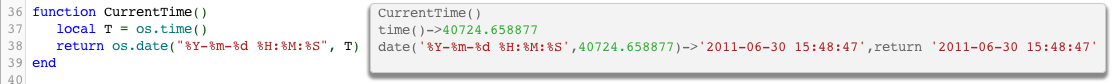# Date/time conversion: Using the fuzzy date/time parser

## Contents

The os.date function is great for outputting arbitrary date/time formats. It’s straightforward to use, here is an example in this case formatting the current time:Here is another example, in this case we parse the input time using the fuzzy date parser and then output it using os.date:You can see how flexible os.date is – especially if you mix it in with using the Lua string library for doing arbitrary string handling.

The formatting codes accepted within the date formatting string are the same as those supported by strftime. This table shows the available codes:

Code Replaced by Example
%a Abbreviated weekday name Thu
%A Full weekday name Thursday
%b Abbreviated month name Aug
%B Full month name August
%c Date and time representation Thu Aug 23 14:55:02 2001
%d Day of the month (01-31) 23
%H Hour in 24h format (00-23) 14
%I Hour in 12h format (01-12) 02
%j Day of the year (001-366) 235
%m Month as a decimal number (01-12) 08
%M Minute (00-59) 55
%p AM or PM designation PM
%S Second (00-61) 02
%U Week number with the first Sunday as the first day of week one (00-53) 33
%w Weekday as a decimal number with Sunday as 0 (0-6) 4
%W Week number with the first Monday as the first day of week one (00-53) 34
%x Date representation * 08/23/01
%X Time representation * 14:55:02
%y Year, last two digits (00-99) 01
%Y Year 2001
%% A % sign %

One useful trick for getting the date/time in a useful format is to use this:

`local T = os.date("*t")`

This returns a Lua table of date/time components, i.e.:For more documentation on os.date and os.time see the Lua reference manual online.

The following code snippet is handy in exercising all the different available formatting codes:

```function CurrentTime()
local F = [[%%a=%a
%%A=%A
%%b=%b
%%B=%B
%%c=%c
%%d=%d
%%H=%H
%%I=%I
%%j=%j
%%m=%m
%%M=%M
%%p=%p
%%S=%S
%%U=%U
%%w=%w
%%W=%W
%%x=%x
%%X=%X
%%y=%y
%%Y=%Y
]]
return os.date(F, os.time())
end```